Question

1)The volume of water needed to dissolve 0.0533 grams of lead iodide is __L.

2)The mass of nickel(II) cyanide that is dissolved in 175 mL of a saturated solution is __ grams.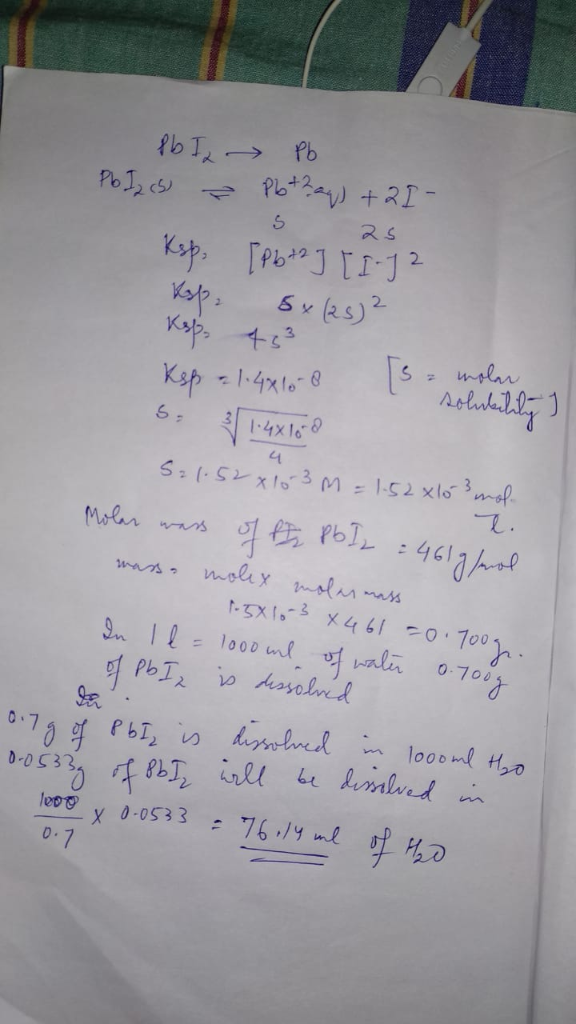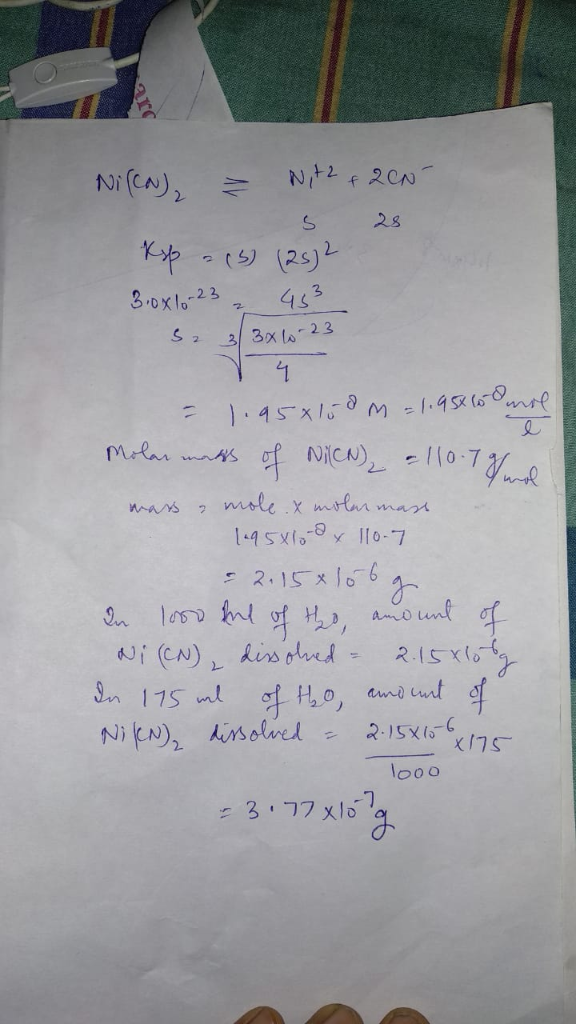#### Earn Coins

Coins can be redeemed for fabulous gifts.

Similar Homework Help Questions
• ### Determine the mass in grams of lead (II) iodide that will dissolve in 500.0 mL of...

Determine the mass in grams of lead (II) iodide that will dissolve in 500.0 mL of a solution containing 1.82 grams of lead (II) nitrate. Ksp of lead (II) iodide is 1.4 x 10-8. Hint: Think about common ion effect.

• ### The mass of nickel(II) hydroxide that is dissolved in 125 mL of a saturated solution is...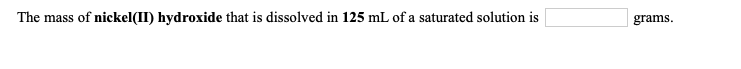The mass of nickel(II) hydroxide that is dissolved in 125 mL of a saturated solution is grams. The volume of water needed to dissolve 0.0547 grams of calcium sulfide is L. Assume no volume change upon addition of the solid.

• ### The mass of nickel(II) hydroxide that is dissolved in 125 mL of a saturated solution is...The mass of nickel(II) hydroxide that is dissolved in 125 mL of a saturated solution is grams. The volume of water needed to dissolve 0.0547 grams of calcium sulfide is L. Assume no volume change upon addition of the solid.

• ### The volume of water needed to dissolve 0.0661 grams of nickel(II) carbonate is L. Assume no...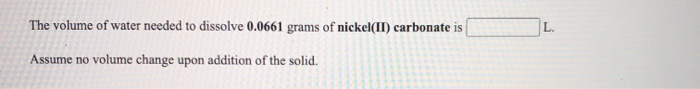The volume of water needed to dissolve 0.0661 grams of nickel(II) carbonate is L. Assume no volume change upon addition of the solid. The volume of water needed to dissolve 0.0709 grams of barium sulfate is L. Assume no volume change upon addition of the solid.

• ### The mass of magnesium hydroxide that is dissolved in 125 mL of a saturated solution is...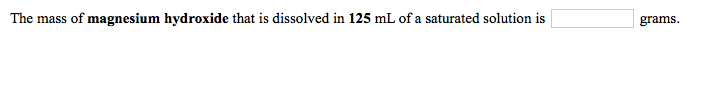The mass of magnesium hydroxide that is dissolved in 125 mL of a saturated solution is grams. The mass of lead carbonate that is dissolved in 150 mL of a saturated solution is grams. The volume of water needed to dissolve 0.0606 grams of calcium chromate is L. Assume no volume change upon addition of the solid.

• ### The mass of calcium hydroxide that is dissolved in 175 mL of a saturated solution is...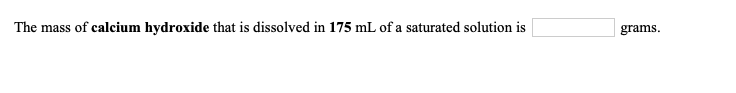The mass of calcium hydroxide that is dissolved in 175 mL of a saturated solution is grams. The volume of water needed to dissolve 0.0522 grams of silver sulfate is L. Assume no volume change upon addition of the solid.

• ### Ksp of PbCO3=1.5 x 10^-13 CaCrO4 7.1 × 10-4 The mass of lead carbonate that is...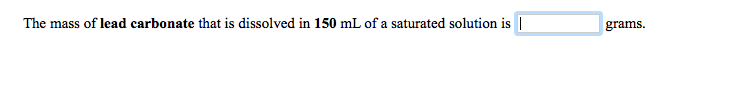Ksp of PbCO3=1.5 x 10^-13 CaCrO4 7.1 × 10-4 The mass of lead carbonate that is dissolved in 150 mL of a saturated solution is | grams. The volume of water needed to dissolve 0.0606 grams of calcium chromate is 0.014 L. Assume no volume change upon addition of the solid.

• ### Use the References to access important values if needed for this question. The volume of water...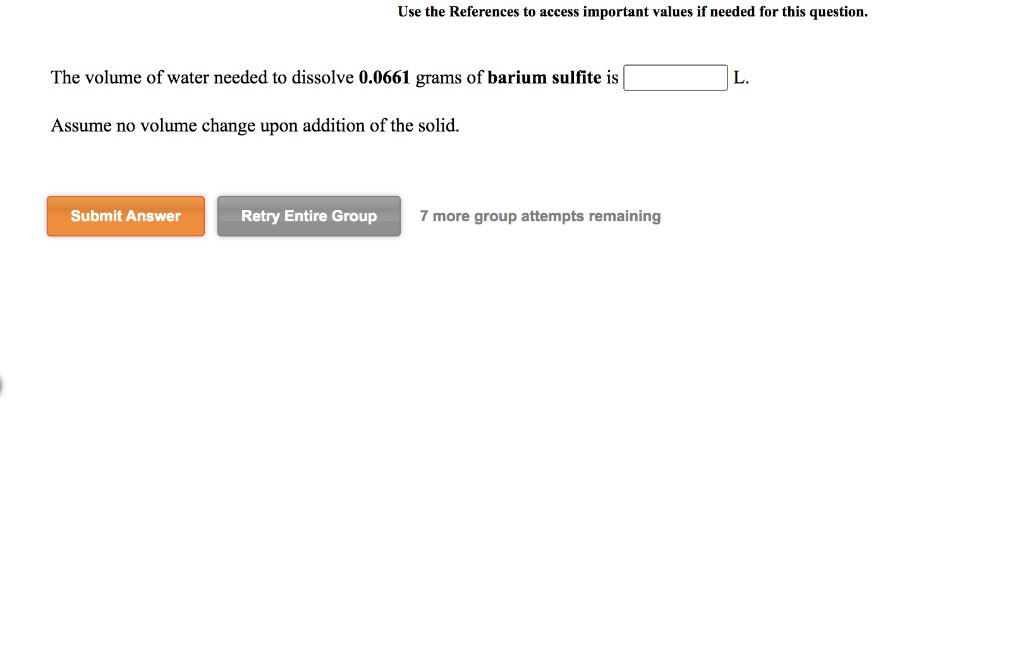Use the References to access important values if needed for this question. The volume of water needed to dissolve 0.0661 grams of barium sulfite is L. Assume no volume change upon addition of the solid. Submit Answer Retry Entire Group 7 more group attempts remaining Use the References to access important values if needed for this question. The mass of cobalt(II) hydroxide that is dissolved in 225 mL of a saturated solution is grams. Submit Answer Retry Entire Group 7...

• ### The mass of nickel(II) cyanide that is dissolved in 150 mL of a saturated solution is...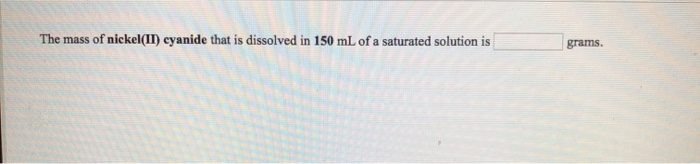The mass of nickel(II) cyanide that is dissolved in 150 mL of a saturated solution is grams.

• ### Values if needed for this question. The mass of cobalt(II) carbonate that is dissolved in 175...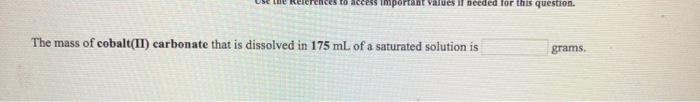Values if needed for this question. The mass of cobalt(II) carbonate that is dissolved in 175 mL of a saturated solution is grams.